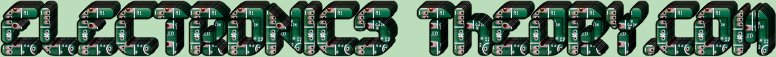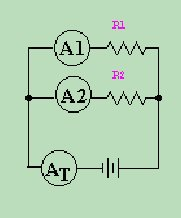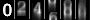# Parallel Circuits - the Plague!

Now that I have you thoroughly confused, let me make things as clear as mud for you. Let's begin with a very simple circuit: 2 resistors and 1 battery. You are given the following information about the circuit:R 1 = 50 Ω
R 2 = 200 Ω
A 1 reads .2 Amps in current ( I=.2 )

Find:
Total Voltage
Total Circuit Resistance
Total Current
And Finally, the Current through A 2

Now this isn't as tuff as it first looks. Let's break the problem down. We know according to Ohm's law, that if we know the resistance and current, we can find the voltage.E R1 = I R1 x R 1 .
E = .2 x 50 = 10
E = 10 Volts.

Now that we know that the voltage for the entire circuit is 10 volts, let's find the total Resistance.
First, we find the reciprocals of the individual resistances:
R 1 = 50 ohms. 1/50 = .02
R 2 = 200 ohms. 1/200 = .005
Now we add the two reciprocals together:
.02 + .005 = .025

Finally we take the reciprocal of the sum:
1 / .025 = 40 Ω

So if the Total Voltage of the circuit is 10 Volts, and the Total Resistance = 40 Ω then by using Ohms Law again we can find the total current.
I Total = E Total / R Total
I = 10/40 = ¼ Ampere.

Almost finished now. So far we know:R 1 = 50 Ω
R 2 = 200 Ω
A 1 reads .2 Amps in current ( I=.2 )
V Total = 10
R Total = 40
and
I Total = ¼

Now we have at least 2 methods by which we can find the current through A 2 .
We know that the Total current is the sum of all the individual leg currents, so if we subtract the current of A 1 from the Total current we get this:
I Total - I 1 = I 2 .25 - .2 = I 2 = .05 Amperes.

The other method would be by using Ohms Law. We know the resistance of R 2 = 200 Ω. We also know that the voltage across R 2 = 10 Volts. Hence:
10 Volts / 200 Ω = .05 Amperes.

Either way, our final result is A 2 = .05 Amps
 (On The Following Indicator... PURPLE will indicate your current location) 1 2 3 4 5 6 7 8 9 10 11 12 13 14 15 16 17 18 19 20 21 22 23 24 25 26 27 28 29 30 31 32 33 34 35 36 37 38 39 40 41 42 43 44 45 46 47 48 49 50 51 52 53 54 55 56 57 58 59 60 61 62 63 64 65 66 67 68 69 70 71 72 73 74 75

[COURSE INDEX] [ELECTRONICS GLOSSARY] [HOME]Otherwise - please click to visit an advertiser so they know you saw their ad!

VISITORS: